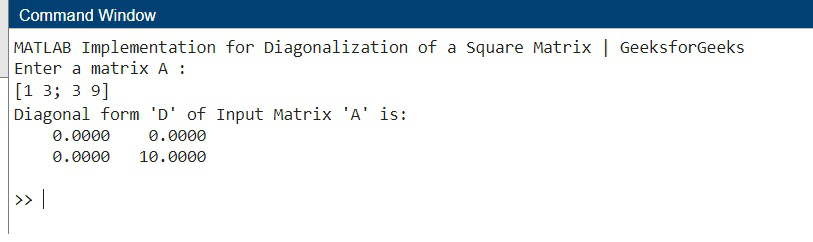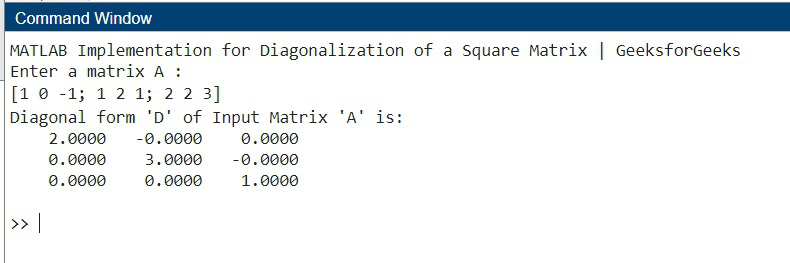# Matrix Diagonalization

Prerequisite: Eigenvalues  and eigenvectors

Let A and B be two matrices of order n. B can be considered similar to A if there exists an invertible matrix P such that B=P^{-1} A P  This is known as Matrix Similarity Transformation.

Diagonalization of a matrix is defined as the process of reducing any matrix A into its diagonal form D. As per the similarity transformation, if the matrix A is related to D, thenand the matrix A is reduced to the diagonal matrix D through another matrix P. Where P is a modal matrix)

Modal matrix: It is a (n x n) matrix that consists of eigen-vectors. It is generally used in the process of diagonalization and similarity transformation.

In simpler words, it is the process of taking a square matrix and converting it into a special type of matrix called a diagonal matrix.

Steps Involved:

Step 1: Initialize the diagonal matrix D as:where λ1, λ2, λ3 -> eigen values

Step 2: Find the eigen values using the equation given below.where, A -> given 3×3 square matrix. I -> identity matrix of size 3×3. λ -> eigen value.

Step 3: Compute the corresponding eigen vectors using the equation given below.where, λi -> eigen value. Xi -> corresponding eigen vector.

Step 4: Create the modal matrix P.Here, all the eigenvectors till Xi have filled column-wise in matrix P.

Step 5: Find P-1 and then use the equation given below to find diagonal matrix D.### Example Problem:

Problem Statement: Assume a 3×3 square matrix A having the following values:Find the diagonal matrix D of A using the diagonalization of the matrix. [ D = P-1AP ]

Solution:
Step 1: Initializing D as:Step 2: Find the eigen values. (or possible values of λ)Step 3: Find the eigen vectors X1, X2, X3 corresponding to the eigen values λ = 1,2,3.Step 5: Creation of modal matrix P. (here, X1, X2, X3 are column vectors)Step 6: Finding P-1 and then putting values in diagonalization of a matrix equation. [D = P-1AP]

We do Step 6 to find out which eigenvalue will replace λ1, λ2, and λ3 in the initial diagonal matrix created in Step 1.We know thatOn solving, we getPutting in the Diagonalization of Matrix equation, we get## Matlab

 % MATLAB Implementation for  % Diagonalization of a Square Matrix: clear all  clc        disp("MATLAB Implementation for Diagonalization   of a Square Matrix | GeeksforGeeks") A = input("Enter a matrix A : ");  [P , D] = eig(A);  D1 = inv(P)*(A)*(P); disp("Diagonal form 'D' of Input Matrix 'A' is:") disp(D1)

Output:

For the Matrix:For the Matrix:Feeling lost in the world of random DSA topics, wasting time without progress? It's time for a change! Join our DSA course, where we'll guide you on an exciting journey to master DSA efficiently and on schedule.
Ready to dive in? Explore our Free Demo Content and join our DSA course, trusted by over 100,000 geeks!